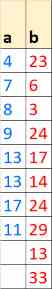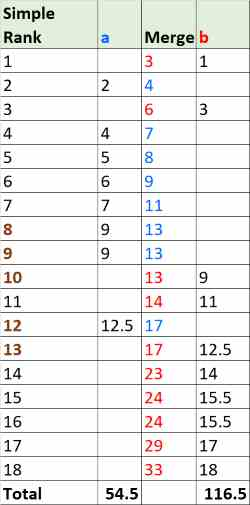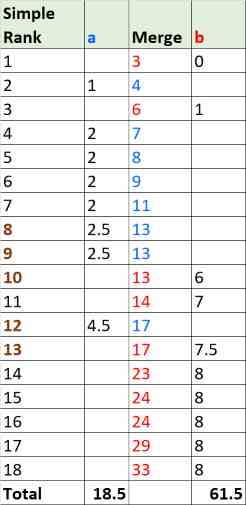# Mann-Whitney U Test(Go to the calculator)

The Mann-Whitney U test is a non-parametric test, it checks continuous or ordinal data for a significant difference between two independent groups. The test merge the data from the two groups. Then, it sorts the data by the value.

## When to use?

The test usually comes as a failsafe option to a Two Sample T-test or Two sample Z-test when the test doesn't meet the normality assumption, or contains many outliers.
A t-test compares the means of the two groups, while a Mann-Whitney U test compares the entire distributions. If the two groups have a similar distribution curve, the test will also compare the medians of the two groups.
A Two Sample T-test is slightly stronger than a Mann-Whitney U Test. A Mann-Whitney U test has 95% efficiency in comparison to a Two sample T-test. If the population is similar to a normal distribution and reasonably symmetric, it is better to use a Two Sample T-test. A Two Sample T-test compares the means of the two groups

• Not Normal, the data is not normally distributes
• Ordinal data, but not interval scaled. You know the order but not the differences between the values.
for example: Unhappy, Neutral, Happy
• Outliers the test is more robust to outliers than t-test

## Assumptions

• Independent observations
• Ordinal / Continuous the compared data consist of ordinal data or continuous data
• Shape the data is not necessarily normally distributed but should have a similar shape. If not you can compare the ranks but not the medians

## Calculate U

• Merge the data from the two groups to one group.
• Sort the data from low value to high value.
• Rank the merged list, the lower value get rank 1 , the second rank 2, etc.
When having ties group, identical value for several observations, the rank will be the average of the ranks for the entire group.
• Calculate the ranks
R1i - the rank of the i member in group 1.
R2i - the rank of the i member in to group 2.
n1 the number of observations in group 1.
n2 the number of observations in group 2.
$$R_1=\sum_{i=1}^{n_1}{R_{1 i}}$$ $$R_2=\sum_{i=1}^{n_2}{R_{2 i}}$$
• Calculate Ui
$$U_1=n_1n2+\frac{n_1(n_1+1)}{2} - R_1.\\ U_2=n_1n2+\frac{n_2(n_2+1)}{2} - R_2.\\ (U_1+U_2=n_1n_2)$$ Since the distribution is symmetrical, usually U is the minimum between U1 and U2. $$U=min(U_1,U_2).$$ It is good for the two tails test, but for the one tail test, it will always assume the following H1: the sample with larger values is bigger than the sample with the smaller values.
In this tool statistics = U2, in this way we can calculate left tail or right tail like any other test.

## Critical Value

When n is small, the tool will use the exact value from tables, the exact critical value is accurate (for common significant levels ), while the p-value is usually interpolated from two values in the table. The tool uses a log interpolation which is more accurate with small p-values and less accurate with large p-values. (the common significant levels are small: 0.05, 0.01).
There is no consensus about what is a small n. When Method="automatic" the tool uses the Exact method when n1 ≤ 25 and n2 ≤ 25,otherwise the tool will use the normal approximation.
When Method="z approximation" the tool uses only the normal approximation

### Statistical tables

Calculated the critical U from a statistical table.

### Corrected normal approximation

To get more accurate results, the tool uses continuity correction and ties corrections. ties is a group of observations with the same value) $$z = \frac { U_2 - \mu_u + C_{continuity}} {\sigma_u}$$ $$\mu= \frac {n_1 n_2} {2}$$ $$\sigma^2= \frac {n_1 n_2(n_1 + n_2 + 1)} {12} (1 - C_{ties})$$

#### Ties correction

$$n = n_1 + n_2 \\ C_{ties} = \sum_{i=1}^{t}{\frac{f_t^3-f_t}{n^3-n}}$$ t - group number of ties.
f_t - number of values in group t.

#### Continuity correction

When using discrete data to continuous distribution it is better to use the c=0.5 continuity correction.
If  U > μ , Ccontinuity = - 0.5
If  U < μ , Ccontinuity = 0.5

When using a continuous data, Ccontinuity = 0.

## Example

In the following example, we check the number of questions answered correctly in two independent groups, one group have completed a training before performed the test while the other group haven't complete the training.
the significant level (α) is 0.05.

Following the test results. The sample sizes: n1=8, n2=10### Indirect method

I prefer the indirect method which I find easier with big samples, and not much more complex with small samples.

• Merge the lists of the two groups to one list.
• Sort by the value, the smallest value first.• Simple Rank - rank by the value, the lower Absolute value get 1 rank, the second 2 etc.
• Rank - usually will be the same as Simple Rank. When the same value repeats, tie, the rank is the average of the simple ranks
The value 13 repeats 3 times.
$$\frac{8+9+10}{3}=9$$ The value 17 repeats 2 times.
$$\frac{12+13}{2}=12.5$$ The value 24 repeats 2 times.
$$\frac{15+16}{2}=15.5$$ R1 = 2 + 4 + 5 + 6 + 7 + 9 + 9 + 12.5 = 54.5
R2 = 1 + 3 +9 + 11 +12.5 + 14 + 15.5 + 15.5 + 17 + 18 = 116.5
• Calculate Ui
$$U_1=n_1n2+\frac{n_1(n_1+1)}{2} - R_1 = 8*10+frac{8*(8+1)}{2} - 54.5 = 61.5 \\ U_2=n_1n2+\frac{n_2(n_2+1)}{2} - R_2 =8*10+\frac{10*(10+1)}{2} - 116.5 = 18.5 \\ (U_1+U_2=61.5 + 18.5 = 80, n_1n_2=8*10 = 80)$$ U = min(61.5 , 18.5) = 18.5

### Direct method

• Merge the lists of the two groups to one list.
• Sort by the value, the smallest value first.• For each value check how manny values from the other group have smaller value.
• Tie - count 0.5 for each value from the other group with the same value.
• Group a (blue)
• Rank2 - there is one red(b) value smaller than 4: 3, fill 1 in column a.
Rank4 - there are two red(b) values smaller than 7: 3,6, fill 2 in column a.
Ranks 5,6,7 - the same like Rank4. Ranks 8,9 - there are two red(b) values smaller than 13: 3,6 and one equal red value, 2 + 0.5 = 2.5, fill 2.5 in column a.
Rank12 - there are 4 red(b) values smaller than 17: 3,6,13,14 and one equal red value, 4 + 0.5 = 4.5, fill 4.5 in column a.
U1 = 1+2+2+2+2+2.5+2.5+4.5 = 18.5
• Group b (red)
• Rank1 - there is no any blue(a) value smaller than 3, fill 0 in column b.
Rank3 - there is one blue(a) value smaller than 6: 4, fill 1 in column b.
Rank10 - there are 5 blue(a) values smaller than 13: 4,7,8,9,11 and 2 equal values, 5 + 2 * 0.5 = 6, fill 6 in column b.
Rank11 - there are 7 blue(a) values smaller than 14: 4,7,8,9,11,13,13 fill 7 in column b.
Rank13 - there are 5 blue(a) values smaller than 17: 4,7,8,9,11,13,13 and one equal value, 7 + 0.5 = 7.5, fill 7.5 in column b.
Ranks 14,15,16,17,18 - there are 8 blue(a) values smaller than 14, all the blue group, fill 8 in column b.
U2 = 0+1+6+7+7.5+8+8+8+8+8 = 61.5

### Statistical tables

#### Two tailed (H0: Group a = Group b)

• Critical Value
Check the the two tails statistic table, for α=0.05, n1=8, n2=10.
The critical U is 17.
• P-value
For α=0.05, critical U is 17.
For α=0.1, critical U is 20.
Since 18.5 is between 17 and 20, the p-value will be between 0.05 and 0.1 .
The tool will do a logarithmic extrapolation: p-value = 0.0707
• Decision
Since p-value > α (0.0707 > 0.05) or alternatively since U > Ucritical (18.5 > 17), accept H0.
• Website
The website uses U2 instead of U.
Left critical U = 17.
Right critical U = n1n2 - 17 = 8 * 10 - 17 = 63.
Since U2 (18.5) is in the following range: [17,63], accept H0. When U2 = 17 or 63 you still accept the H0.

#### Left tail (H0: Group a ≥ Group b)

• Critical Value
Check the the two tails statistic table, for α = 2 * 0.05 = 0.1, n1=8, n2=10.
The critical U is 20.
• P-value
P-value = p-value(Two tailed) / 2 = 0.0707 / 2 = 0.0354
• Decision
Since p-value < α (0.0354 < 0.05) or alternatively since U2 < Ucritical (18.5 < 20), reject H0.

#### Right tail (H0: Group a < Group b)

• Critical Value
Check the the two tails statistic table, for α = 2 * 0.05 = 0.1, n1=8, n2=10.
The value in the table is 20.
The critical U is n_1n_2 - value from the table = 8 * 10 - 20 = 60.
• P-value
P-value = 1 - p-value(Two tailed) / 2 = 1 - 0.0707 / 2 = 0.9646
• Decision
Since p-value > α (0.9646 > 0.05) or alternatively since U2 < Ucritical (18.5 < 60), reject H0.

### Corrected normal approximation

• $$group_1: [13,13,13], \quad f_1=3.\\ group_2: [17,17], \quad f_2=2.\\ group_3: [24,24], \quad f_3=2.$$ There are 3 tie groups (t=3):
$$n=n_1+n_2=8+10=18. \\ C_{ties} = \sum_{i=1}^{t}{\frac{f_t^3-f_t}{n^3-n}} = {\frac{3^3-3+2^3-2+2^3-2}{18^3-18}}=\frac{36}{5814}=\frac{2}{323}=0.00619$$
• $$\mu_u= \frac {n_1n_2}{2}=\frac {8*10}{2}=40$$ $$\sigma_u^2= \frac {n_1 n_2(n_1 + n_2 + 1)} {12} (1 - C_{ties}) = \frac {8*10(8 + 10 + 1)} {12} (1 - 0.00619) = 125.8826, \sigma_u = 11.22$$ Since the data is discrete and U2 < μ , Ccontinuity = 0.5. $$Z = \frac { U_2 - \mu_u + C_{continuity}} {\sigma_w} = \frac { 18.5 - 40 + 0.5} {11.22} = -1.872$$
• P( z ≤ Z) = P( z ≤ -1.872) = 0.0306

#### Two tailed (H0: Group a = Group b)

• P-value = 2 * 0.0306 = 0.0612
• Since 0.0612 > 0.05, accept H0.

#### Left tail (H0: Group a ≥ Group b)

• P-value = P( z ≤ -1.872) = 0.0306
• Since 0.0306 < 0.05, reject H0.

#### Right tail (H0: Group a < Group b)

• P-value = P( z ≥ -1.872) = 1 - P( z ≤ -1.872) = 1 - 0.0306 = 0.9694
• Since 0.9604 > 0.05, accept H0.